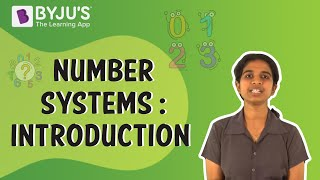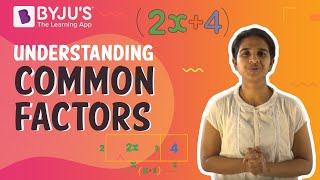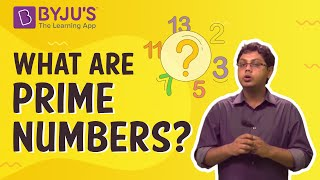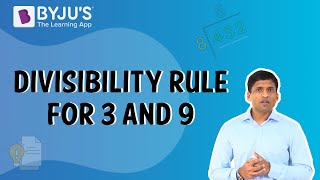# Playing with numbers Class 6 notes - Chapter 3

A number is defined as an arithmetical value, expressed by a word, symbol, and figures. These numbers can be written in single digits, double digits, three-digits in the generalized form.

## Types of Numbers

A number system is a system of writing for expressing numbers. According to the number system, the different types of a number includes:

• Prime numbers
• Even numbers
• Odd numbers
• Whole numbers
• Natural numbers
• Composite numbers

#### For More Information On Number Systems, Watch The Below Video.To know more about Different Types of Numbers, visit here.
Lets us look into some solved example problems.

Write all the factors of 65
65 is a composite number.
65 = 1 × 65
5 x 13 = 65
Factors of 65: 1, 5, 13, 65.
Find the common factors of: 850 and 680
The common factors of 850 and 680 are 2, 5 and 17.

## Facts About Factors and Multiples

### Factors

A factor of a number is an exact divisor of that number.

Example: 1, 2, 3, and 6 are the factors of 6.

#### For More Information On Factors and Common Factors, Watch The Below Video.### Properties of factors

Properties of factors of a number:

• 1 is a factor of every number.
• Every number is a factor of itself.
• Every factor of a number is an exact divisor of that number.
• Every factor is less than or equal to the given number.
• Number of factors of a given number are finite.

To know more about Factors and Mutiples, visit here.

### Perfect numbers

A number for which sum of all its factors is equal to twice the number is called a perfect number.

Example: Factors of 28 are 1, 2, 4, 7, 14 and 28.

Here, 1 + 2 + 4 + 7 + 14 + 28 = 56 = 2 × 28
Therefore, sum of factors of 28 is equal to twice the number 28.
To know more about Perfect Numbers, visit here.

### Multiples

Multiples of a number are those numbers which we get on multiplying the number by any integer.
Example: Multiples of 3 are 6, 9, 12, 15, 18 etc.

### Properties of multiples

Properties of multiples of a number:

• Every multiple of a number is greater than or equal to that number.
• Number of multiples of a given number is infinite.
• Every number is a multiple of itself.

## Ones with the One and the Others

### Prime numbers

Numbers other than 1 whose only factors are 1 and the number itself are called Prime numbers.

Example: 2, 3, 5, 7 etc.

#### For More Information On Prime Numbers, Watch The Below Video.To know more about Prime Numbers, visit here.

### Composite numbers

Numbers having more than two factors are called Composite numbers.

Example: 4, 6, 8 etc.
To know more about Composite Numbers, visit here.

## Divisible by 2 or 5 or Both

### Divisibility Tests

A divisibility rule is a method of determining whether a given integer is divisible by a fixed divisor without performing division, usually by examining its digits.
We have divisibility rules for 2, 3, 4, 5, 6, 7, 8, 9, 10 and 11.

### Divisibility tests for 2

If one’s digit of a number is 0,2,4,6 or 8, then the number is divisible by 2.
Example: 12, 34, 56 and 78.

### Divisibility tests for 4

A number with 3 or more digits is divisible by 4 if the number formed by its last two digits (i.e. ones and tens) is divisible by 4.
Example: 1396 is divisible by 4 since its last two digits i.e. 36 is divisible by 4.

### Divisibility tests for 3

A number is divisible by 3, if sum of its digits is divisible by 3.
Example: Take 27.
Sum of its digits = 2+7= 9, which is divisible by 3.
Therefore, 27 is divisible by 3.

#### For More Information On Divisibility Tests, Watch The Below Video.### Divisibility tests for 5

If the one’s digit of a number is either 5 or 0, then it is divisible by 5.
Example: 75, 90, 100 and 125.

### Divisibility tests for 8

A number with 4 or more digits is divisible by 8, if the number formed by its last three digits is divisible by 8.
Example: 73512 is divisible by 8 since its last three digits i.e. 512 is divisible by 8.

### Divisibility tests for 6

If a number is divisible by 2 and 3 both, then it is divisible by 6 also.
Example: 120 is divisible by 2 and 3. Therefore, it is divisible by 6 also.

### Divisibility tests for 7

Double the last digit and subtract it from the remaining leading cut number. If result is divisible by 7, then the original number is divisible by 7. Example: 826 is divisible by 7 since, 82 – (6 × 2) = 82 – 12 =70, which is divisible by 7.

### Divisibility tests for 9

A number is divisible by 9 if sum of its digits is divisible by 9.
Example: Consider 126.
Sum of its digits = 1+2+6 = 9, which is divisible by 9.
Therefore, 126 is divisible by 9.

### Divisibility tests for 11

Find difference between sum of digits at odd places (from the right) and sum of digits at even places (from the right) of a number. If the difference is either 0 or divisible by 11, then the number is divisible by 11.
Example: 1234321 is divisible by 11 since, (1+3+3+1) – (2+4+2) = 8 – 8 = 0, which is divisible by 11.

### Divisibility tests for 10

If one’s digit of a number is 0, then the number is divisible by 10.
Example: 10, 20, 30 and 40.
To know more about Divisibility Rules, visit here.

### Common factors

The factors of 4 are 1, 2 and 4.
The factors of 18 are 1, 2, 3, 6, 9 and 18.
The numbers 1 and 2 are common factors of both 4 and 18.

#### For More Information On Common Factors, Watch The Below Video.### Common multiples

Multiples of 3 are 3, 6, 9, 12, 15, 18,….
Multiples of 5 are 5, 10, 15, 20, 25, 30,…
Multiples of 6 are 6, 12, 18, 24, 30, 36,…
Therefore, common multiples of 3, 5 and 6 are 30, 60,….

## The Prime Factor

### Prime Factorization

When a number is expressed as a product of prime numbers, factorisation is called prime factorisation.

Example: Prime factorisation of 36 is 2×2×3×3.
To know more about Prime Factors, visit here.

Learn more about the numbers from the topics given below: